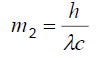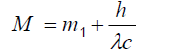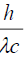Parası kalmadığı için otobüse binemiyordur ailesi porno izle ona daha yeni para gönderdiği için tekrar porno istemeye utanınca mecburen otostop çekmek için youporn çantasını alarak yol kenarına gelir etekli porno liseli türk kız yol kenarında dururken yanına yaklaşan porno kibar bir gencin onu gideceği yere kadar bırakmak porno izle istemesine çok mutlu olur arabaya bindiklerinde gideceği yer ile porno arabayı kullanan adamın gittiği yer arasında çok mesafe sex izle farkı olduğunu anlayan türk kız bu yaptığı porno indir iyilik karşısında arabada ona memelerini açar porno sapıklaşan adam yol kenarındaki hotelde durarak porno izle üniversiteli otostop çeken türk kızına odada sakso çektirip sikerThe Waved Space
All submissions of the EM system will be redirected to Online Manuscript Submission System. Authors are requested to submit articles directly to Online Manuscript Submission System of respective journal.

Viewpoint
, Volume: 6( 3)

# The Waved Space

*Correspondence:
Maziar Naderi , Environmental health engineering, Tehran University of Medical Sciences, Iran, E-Mail: [email protected]

Received: July 31, 2018; Accepted: August 16, 2018; Published: August 23, 2018

Citation: Naderi M. The Waved Space. J Phys Astron. 2018; 6(3):162.

### Introduction

For centuries, nature of the space and the material that has filled it has attracted the minds of many scientists and physicists. This article describes the theory of emergence of the universe and structure of the space. According to this theory, since time is not separated from space, time has always existed and has no starting point, but the emergence of matter has been the starting point. In general, we must say that: 1) the space that forms our universe is a corrugated space; 2) the direction of motion of any particle moving in this space is also a waved path with a certain wavelength; 3) the space that compresses and shortens its wavelength turns into matter.

Scientists have long sought to find the starting point of space and time. The human mind thinks about the moment, in which the formation of space and time started, but the universe does not understand such thinking. In this article, theory of the emergence of the universe and the structure of space would be briefly described. According to this theory, space and time do not have a starting point. Space has always existed, and since time is not separated from space, time has also existed always and has no starting point, but the emergence of matter has been the starting point. According to this theory, before the matter occurred, the space had a uniform structure (with layers or parallel lines). This space slowly begins to shrink and collapse in all directions. As a result of this contraction, the space becomes curved, and as the contraction increases, the wavelength of the space becomes shorter and the wave amplitude becomes longer. The nature of these waves is energy.

Based on the well-known equation of Einstein, energy changes are proportional to mass changes.Space accumulation, resulting in shorter space wavelength, increases energy. This increase in energy causes the formation of mass. This relation is observed in the Einstein equation.

E=mc2

At a point in the space where this wave is condensed and the wavelength is very short, there is an enormous amount of energy at that point. As a result, suddenly a dense material has been formed, and an explosion has occurred and the particles are scattered around. Just like the water that contains many ions and salts, but because they are soluble in water, we do not see them, and when the physical conditions of the solution change, crystallization and precipitation occur, and as a result, we see the particles. The components that we originally thought were not uniform in the water, but particles emerge with internal energy changes.

With regard to this theory, the shorter wavelength of space generates matter, and the matter is the dense space with very short wavelengths. The relation between mass and wavelengths is shown in the de Broglie formula.

λ = h/mv

This equation shows that every particle with the mass ‘m’ has a waved motion and this wavelength is computable. According to this equation, mass is related to wavelength. Hence, as the mass increases, the wavelength of the object motion becomes shorter.

For example, the direction of movement of the planet Earth around the Sun is a curved path, which according to this formula has a wavelength of about 3.3 mm. Based on this theory, this wavelength other than the wavelength of the object's path may be the intrinsic wavelength of the object.

Because, according to this theory, the corrugation of space causes the formation of matter. The wavelength of these waves is shorter in the center of the sphere and is longer in the outer layer of matter.

For example, the planet Earth is a mass that is formed by the explosion and has been transformed over time. The wavelength is shorter in the center of the planet and in its outer cortex which forms the Earth's atmosphere, is longer.

The matter formed by this slow contraction was a pure matter. Due to the sudden expansion of the pure matter, various materials were formed with special wavelengths. These materials were the same chemical elements with different wavelengths.

Now, based on this theory, we explain the increase in the particle mass that moves at a speed near the speed of light.Based on this formula, as the kinetic energy of a particle that moves at a speed near the speed of light increases, the mass of the particle enhances. The amount of added mass is. But when the particle stops, the mass is the same as the original mass. So where does this extra mass come from?

This increase in particle mass can be explained by the theory of waved space. In general, we must say that: 1) the space that forms our universe is a corrugated space; 2) the direction of motion of any particle moving in this space is also a waved path with a certain wavelength; 3) The space that compresses and shortens its wavelength turns into matter.

When a particle moves in space, in accordance with the second principle, the path of motion is waved, and if it moves with the speed of light, the particle's wavelength is equal to the wavelength of light (10-6 microns). As a result, this particle reduces the wavelength of space. And this shortening of the wavelength of space generates mass, and this is the mass produced by the motion of a particle at the speed of light, which is added to the initial mass of the particle, and when the particle is stopped, the wavelength of the space returns to its original value, and the mass of the space occupied by the particle is zero.C is the speed of light or particle, v is the amount of space occupied by the particle, λ is the wavelength of the particle or the wavelength of space occupied by the particle, h is Planck constant, m2 is mass added to the particle mass and m1 is particle mass.

When the particle stops,is zero and the particle has the same initial mass. By the theory, the gravitational redshift can be explained. Around the giant bodies and areas with high gravity such as block hole, the space is more compact and the wavelength of space is shorter than the space far away. So the wavelength reaches out to our eyes tends to be red, because the space around the observer who sees the light has a wavelength longer since electromagnetic waves spread along the waved space.

In addition, by the theory, many natural phenomena and many physics challenges, such as the generation and emission of electromagnetic waves, the absorption spectra and elemental emission, the structure of matter, the nature of light and gravitational waves, subatomic particles wave functions, and many other phenomena in quantum physics can be explained.

Welcome Message

###### Citations : 243

Journal of Physics & Astronomy received 243 citations as per Google Scholar report

#### Indexed In

• Open J Gate
• China National Knowledge Infrastructure (CNKI)
• Cosmos IF
• Directory of Research Journal Indexing (DRJI)
• MIAR
• Secret Search Engine Labs
• Euro Pub